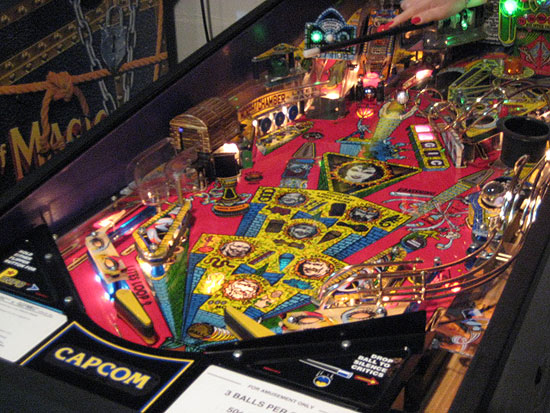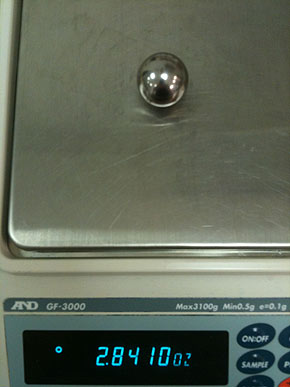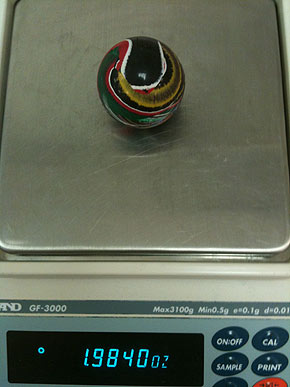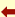DEFYING THE LAWS OF PHYSICS

We know why an actual pinball rebounds around a pinball machine playfield; because we hit it with a flipper bat.A batted ball

Have you ever dropped an actual pinball onto a hard floor and had it bounce back up towards you? Why does a solid metal pinball “bounce” back up to us, just like a rubber ball, when dropped? This action seems to defy the laws of physics; yet it does not. The reason is simple. That reason, “For every action, there is an equal and opposite reaction.” Read more to learn a slightly deeper explanation.

History

Contrary to a belief held by some, it was Galileo and not Newton, who discovered that two things of different mass will fall at the same rate. However, we will use Newton’s math to explain why this happens. Maybe this is why some people get confused?

The Math (Newton’s second law of motion)
Force equals mass times acceleration.
F = m x aSolid massBouncy mass

The Terms
F = Force
For this example, think of force as energy.

m = mass
For our purposes, think of mass as weight.

a = acceleration
In this article we will be equating acceleration to speed. Acceleration here is a constant; the rate which gravity pulls an object to the floor. That rate of constant acceleration is about 32 Ft/Sec/Sec, or expressed a little differently with metric units 9.8 m/s².

When playing pinball, you can effectively see gravity’s influence when you flip the ball from the lower part of the playfield up to the back of the inclined playfield. And, if you watch carefully, you can see that the ball accelerates as it races down back towards the flippers. This falling back towards the flippers is the constant effect of gravity.

Potential Energy versus Kinetic Energy

A second consideration is the energy of the system. We are equating force as energy.

Let’s examine the difference between potential energy and kinetic energy by use of a rubber band (elastic band).

The Terms
Potential Energy
Consider this the stored energy.

Kinetic Energy
Consider this the “extra” energy due to motion.

So, getting back to our example of a rubber band, the physical make up of the band itself stores energy in the rubber of which it is made. Then, you add energy by holding one spot in the band and pulling the opposite side back. You can feel this extra energy as you pull. And, the further you move back the band, the more energy you feel.

Moving to our example of two dropped balls, the bodies of the balls store energy. When we lift the balls up off of the table, we are moving the balls and thus imparting more energy.

Another Physics Law
(Newton’s third law of motion)
For every action, there is an equal and opposite reaction. This is where we started.

This law is shown when a ball hits the floor. The floor effectively “pushes back” in the opposite direction. The original direction is towards the floor. The opposite direction is back towards the starting point.

All Together

So to summarize, we have two weighty spheres. We give them energy by raising them above the floor. Then we accelerate them by taking advantage of gravity as we let them fall.

The Rebound

OK, now for the second part, the collision with the floor. From the video above you can see that both balls bounce back up toward where they started. Why is this? Well, in both cases, the floor effectively “pushed” back on the balls with the same force applied to them.

In the case of the rubber ball, it bounces back because it has an elastic collision with the floor. The inside portion of the ball acts like a rubber band. One that gets pushed (pulled in reverse), by the force created from both the fall and gaining potential energy. That new total energy then gets transferred into an equal and opposite reaction of movement back in the reverse direction from which it came.

As per the metal pinball, it bounces back because it too has a collision with the floor. In the case of this solid metal ball, the material inside the ball cannot compress and transfer the extra energy somewhere inside the ball. Therefore the energy must be used in another way. That means is for the ball to rebound back toward its starting position; thusly seeking a point of equilibrium.

In summary, the rubber ball gets its internal rubber band energized and it is that transferred energy which is released to raise the ball back up toward its starting position.

Whereas the metal ball has no internal rubber band. In fact there is only metal inside the ball. This metal cannot easily be compressed. So, only the equal and opposite force of the floor pushes the ball back toward its original starting position.

Final Question

One final question; did you ever try to replace the metal pinball inside your machine with a rubber ball?Back to the learn page